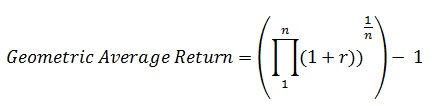# Average rate of return (ARR)

Posted in Finance, Accounting and Economics Terms, Total Reads: 5257

## Definition: Average rate of return (ARR)

Rate of return or return on investment is the amount of money gained or lost on an investment. Mathematically, it is calculated by dividing the gain/loss by the amount of investment and is generally expressed as a percentage.

Average rate of return is generally relevant when we consider the return on an investment over multiple periods. The average rate of return can further be of two types:

1. Arithmetic Average Return: This is the arithmetic average of returns over individual periods.

2. Geometric Average Return: This is like the geometric mean of all the returns over all individual periods.

Mathematically, it is calculated as:Where

r = Average in each of the periods

n = No. of Periods

Browse the definition and meaning of more terms similar to Average rate of return (ARR). The Management Dictionary covers over 7000 business concepts from 6 categories. This definition and concept has been researched & authored by our Business Concepts Team members.

Search & Explore : Management Dictionary

Similar Definitions from same Category: